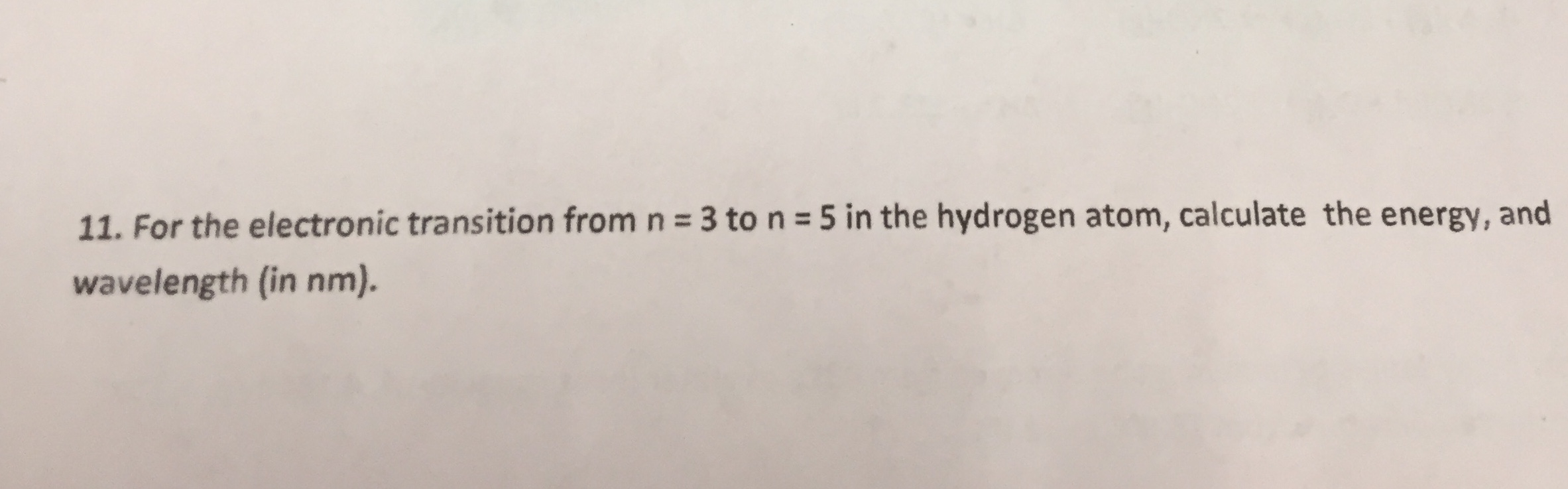11. For the electronic transition from n 3 ton 5 in the hydrogen atom, calculate the energy, andwavelength (in nm).

Questionhelp_outlineImage Transcriptionclose11. For the electronic transition from n 3 ton 5 in the hydrogen atom, calculate the energy, and wavelength (in nm). fullscreen
Step 1

The energy of the electronic transition is calculated by below equation,

Step 2

The electronic transition occurs from n= 3 to n=5.

Energy of the n= 3 to n=5 transition is,

Step 3

The wavelength of the electronic transitio...

Want to see the full answer?

See Solution

Want to see this answer and more?

Our solutions are written by experts, many with advanced degrees, and available 24/7

See Solution
Tagged in

Quantum mechanics